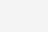# C语言结构体里的成员数组和指针```final void retryUpdate(long x, HashCode hc, boolean wasUncontended) {
int h = hc.code;
boolean collide = false;                // True if last slot nonempty
for (;;) {
Cell[] as; Cell a; int n; long v;
if ((as = cells) != null && (n = as.length) > 0) {// 分支1
if ((a = as[(n - 1) & h]) == null) {
if (busy == 0) {            // Try to attach new Cell
Cell r = new Cell(x);   // Optimistically create
if (busy == 0 && casBusy()) {
#include <stdio.h>
struct str{
int len;
char s;
};

struct foo {
struct str *a;
};

int main(int argc, char** argv) {
struct foo f={0};
if (f.a->s) {
printf( f.a->s);
}
return 0;
}```

`printf("%x\n", f.a->s);`

1）为什么不是 13行if语句出错？f.a被初始化为空了嘛，用空指针访问成员变量为什么不crash？

2）为什么会访问到了0x4的地址？靠，4是怎么出来的？

3）代码中的第4行，char s 是个什么东西？零长度的数组？为什么要这样玩？

#### 结构体中的成员

```struct test{
int i;
char *p;
};```

`struct test t;`

```# t实例中的p就是一个野指针
(gdb) p t
\$1 = {i = 0, c = 0 '00', d = 0 '00', p = 0x4003e0 "1\355I\211\..."}

# 输出t的地址
(gdb) p &t
\$2 = (struct test *) 0x7fffffffe5f0

#输出(t.i)的地址
(gdb) p &(t.i)
\$3 = (char **) 0x7fffffffe5f0

#输出(t.p)的地址
(gdb) p &(t.p)
\$4 = (char **) 0x7fffffffe5f4```

```struct test{
int i;
short c;
char *p;
};

int main(){
struct test *pt=NULL;
return 0;
}```

```(gdb) p pt
\$1 = (struct test *) 0x0
(gdb) p pt->i
Cannot access memory at address 0x0
(gdb) p pt->c
Cannot access memory at address 0x4
(gdb) p pt->p
Cannot access memory at address 0x8```

#### 指针和数组的差别

• 对于char s来说，汇编代码用了lea指令，lea   0x04(%rax),   %rdx

• 对于char*s来说，汇编代码用了mov指令，mov 0x04(%rax),   %rdx

```struct test{
int i;
short c;
char *p;
char s;
};

int main(){
struct test *pt=NULL;
printf("&s = %x\n", pt->s); //等价于 printf("%x\n", &(pt->s) );
printf("&i = %x\n", &pt->i); //因为操作符优先级，我没有写成&(pt->i)
printf("&c = %x\n", &pt->c);
printf("&p = %x\n", &pt->p);
return 0;
}```

#### 关于零长度的数组

```#include <stdlib.h>
#include <string.h>

struct line {
int length;
char contents; // C99的玩法是：char contents[]; 没有指定数组长度
};

int main(){
int this_length=10;
struct line *thisline = (struct line *)
malloc (sizeof (struct line) + this_length);
thisline->length = this_length;
memset(thisline->contents, 'a', this_length);
return 0;
}```

```(gdb) p thisline
\$1 = (struct line *) 0x601010

(gdb) p *thisline
\$2 = {length = 10, contents = 0x601010 "\n"}

(gdb) p thisline->contents
\$3 = 0x601014 "aaaaaaaaaa"```

```struct line {
int length;
char *contents;
};

int main(){
int this_length=10;
struct line *thisline = (struct line *)malloc (sizeof (struct line));
thisline->contents = (char*) malloc( sizeof(char) * this_length );
thisline->length = this_length;
memset(thisline->contents, 'a', this_length);
return 0;
}```

```(gdb) x /14b thisline
0x601010:       10      0       0       0       97      97      97      97
0x601018:       97      97      97      97      97      97```

```(gdb) x /16b thisline
0x601010:       1       0       0       0       0       0       0       0
0x601018:       32      16      96      0       0       0       0       0
(gdb) x /10b this->contents
0x601020:       97      97      97      97      97      97      97      97
0x601028:       97      97```

• 第一行前四个字节是 int length，第一行的后四个字节是对齐。

• 第二行是char* contents，64位系统指针8个长度，他的值是0x20 0x10 0x60 也就是0x601020。

• 第三行和第四行是char* contents指向的内容。

#### 后记

1）看过这篇文章，你觉得C复杂吗？我觉得并不简单。某些地方的复杂程度不亚于C++。

2）那些学不好C++的人一定是连C都学不好的人。连C都没学好，你们根本没有资格鄙视C++。

3）当你们在说有坑的时候，你得问一下自己，是真有坑还是自己的学习能力上出了问题。